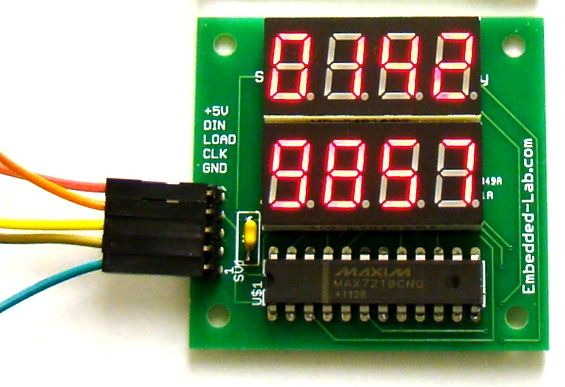How to use an RGB LED – Arduino Tutorial

An RGB LED has 4 pins, one for each color (Red, Green, Blue) and a common cathode. It has tree different color-emitting diodes that can be combined to create all sorts of color! Any color is possible depending on how bright each diode is.

In this tutorial you will learn how to use an RGB LED with Arduino and create unique color combinations.

So, let’s get started!

Step 1: What you will need

For this tutorial you will need:

• Arduino uno
• RGB LED
• 3x 330Ohm resistors

Step 2: The Circuit

The connections are pretty easy, see the image above with the breadboard circuit schematic.

Step 3: The Code

Here’s the code, embedded using codebender!

We will use code from SparkFun Inventor’s Kit – SIK Guide, Example sketch 03. This is the best sketch to learn how an RGB LED works. The code is pretty self-explanatory, and the comments do a better job than me explaining how it works.

For more detail: How to use an RGB LED – Arduino Tutorial

READ  CCS TOGGLE LED EXAMPLE PIC16F877